------------ CCP4 Newsletter - January 1997 ------------

Back to Contents....

# Simplified error estimation a la Cruickshank in macromolecular crystallography

### Garib N. Murshudov and Eleanor J. Dodson

Chemistry Department, University of York, Heslington, York, U.K.

## 1. Introduction

An important part of protein crystallography is refining the fit of model parameters to experimental data. It is intuitively obvious that a model will be more reliable if there are more observations to fit it to, and that some parts of a macromolecular model are more accurately described than others. It is not always easy to parameterise this, but without giving some estimate of the reliability of the model parameters the refinement procedure cannot be complete.

When parameters are estimated by least-squares or maximum likelihood methods their reliability can be estimated from the inverse of the matrix of the second derivatives (see for example Stuart & Ord, 1991). Figure 1. However it is extremely time consuming to both generate and to invert the matrix of second derivatives for many parameters. To our knowledge the only refinement program which has an option to do this, and thus give standard uncertainties of parameters is SHELXL (Sheldrick 1995).

As a community we have been extremely lucky to have interested Durward Cruickshank in this problem. He was instrumental in developing much of the solid theoretical basis for the refinement of small molecules during the 50s and 60s, and has recently addressed the special problems of macromolecules, where there is less reliable data, the range of precision within any given structure is much greater, and the computing problems are still formidable. He points out that protein crystallographers often use somewhat misleading methods to estimate reliability (Cruickshank 1996). One of them is to use the Luzzati plot to assign an overall average error for atomic coordinates. But Luzzati's classic paper (1952) describes the probability distribution of structure factors and does not claim to indicate the reliability of parameters. It is dependent on weights used in refinement. The sigmaA plot described by Read (1986) is also based on a similar distribution. A second method is to use B-values as an indicator of the reliability of atomic positions. As expected, it is easy to demonstrate that there is a relationship between B-value and estimated standard uncertainties (e.s.u.) of atoms but it is important to remember that the B-value is an estimation of atomic mobility but not its reliability.

However approximate standard uncertainties can be obtained from the diagonal terms alone of the second derivative matrix. These can be estimated during the course of refinement, and it is trivial to carry out the matrix inversion of a diagonal matrix. (Murshudov, Vagin & Dodson, 1997):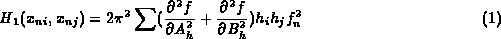where f is residual used for refinement (it could be least-squares or maximum likelihood residuals), A_h and B_h are real and imaginary parts of structure factor, f_n is atomic form factor, x_n is positional parameter.

For B-values:B_n is atomic B-value.

In the following sections we will give Cruickshank's equation for a dispersion precision indicator (DPI) and its modification to utilise R_{free}, and extend them to give some simple equations for DPIs corresponding to approximate e.s.u.-s of the individual atomic coordinates and B-values. The equations for B-value dependent e.s.u. are similar to the equations given by Cruickshank (1949a, 1949b)

## 2. Overall standard uncertainties based on R-value and free R-value

Using equation (1) for orthogonal coordinates and making several simplifications Cruickshank (1960, 1996) gives following equation for an overall dispersion precision indicator (DPI):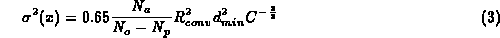where C is completeness, R_{conv} is conventional R-value, d_{min} maximum resolution, N_a number of atoms included in refinement, N_o is number of observations, N_p is number of parameters refined.

He suggests replacing the factor 0.65 by 1.0 as a matter of caution since in the derivation of above equation only diagonal terms of the second derivative matrix are used. This equation does not take into account the effect of geometric restraints and cannot be used at low resolution when N_o - N_p is negative.

If we will assume that R_{free} is the expected value of R and use the relation between them suggested by Cruickshank during the Refinement Workshop reported in Dodson, Kleywegt, Wilson (1996).then we can base DPI on R_{free}Since R_{free} is dependent to some extent on the information about restraints and on the parameterisation used for refinement the equations should be meaningful in all cases (it does not matter if you have refined with or without NCS, isotropic anisotropic or overall B-value). But the equations can only give overall DPI, and cannot indicate the relative precision of different parts of a structure.

To test the agreement between equations (3) and (4) we used catalase from Micrococcus lysodeikticus refined at three different resolutions 1.5, 1.83 and 1.96A (Murshudov et al 1997). For the structure refined at 1.5A R_{conv} and R_{free} are 11.7 and 14.0%, suggesting DPI-s of 0.045A and 0.048A respectively. For the structure refined at 1.83A R_{conv} and R_{free} are 11.8 and 15.0 % giving DPI-s of 0.082A and 0.086A, while for the 1.96A structure R_{conv} and R_{free} are 16.7 % and 22.7 % with DPI-s of 0.143A and 0.147A respectively. This close agreement show that at medium and high resolution DPI-s can be derived from the R_{free} values quite accurately.

Both these equation can only be used sensibly at the end of refinement, when the parameter is near its minimum value, (see Figure 1) and with the assumption that the model is complete. The DPIs are nonsense initially. To demonstrate this: take the extreme case. If the data is complete to 1.5A resolution, but the model consists of random atoms N_a / N_o approx 0.05, R_{free} approx 0.58, and hence DPI approx 0.13 which clearly is not a measure of the precision of the positional parameters.

## 3. Approximation to standard uncertainties of individual atomic parameters

Using equation (1) and the approximation: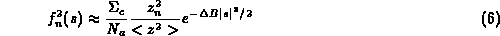then for the B-value dependent e.s.u we can write: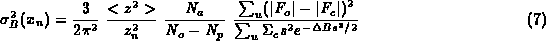where <z²> is average of square of number of electrons, z_n² is square of number of electrons for given atom, delta-B is difference between the Wilson and this atom's B-value, N_o, N_p are defined above, s is reciprocal space vector, F_o and F_c observed and calculated amplitudes of structure factors, Sigma_c the normalisation factor for calculated structure factors, sum_u the summation over the reflections included in refinement.

To avoid negative differences between N_o-N_p we can replace sum_u(|F_o|-|F_c|)²/(N_o-N_p) by sum_f(F_o-F_c)²/N_{free}:where N_{free} is number of `free' reflections and sum_f is the summation over these.

The same approach could be used for approximate e.s.u. of B-valuesor: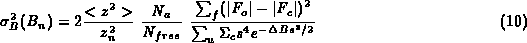Again these equations should be used only at the end stages of refinement, and then Sigma_c could be replaced by Sigma_o and even by |F_o|.

These equations show that the e.s.u. of both positional and thermal parameters will depend on completeness of data, which is expressed through the summation, on the B-value of the atom, and on the agreement between observed and calculated structure factors. More reliable values may be obtained by using a weighted sum over the reflections. Equation (7) and (9) can only be used at high resolution, but equation (8) and (10) could be used at any resolution since they do not involve N_o-N_p. Moreover since equations (8) and (10) use only the agreement of the `free' reflections, the effect of restraints will be incorporated in the estimate.

Note that these approximations are very rough. They could be improved but the effect of the unconsidered non-diagonal terms is expected to be much larger than the effect of approximations and these equations can be used for qualitive reliability assessment.

Again we used the catalase structures for testing. Figure 2-4 shows B-value dependence of the e.s.u. for the positional and thermal parameters. At 1.5A resolution the e.s.u. based on `used' and `free' reflections are very close to each other. At lower resolution this is not so, probably because the `free' reflections contain information about restraints whereas `used' reflection do not know about them.

## 4. Likelihood based DPI

Using maximum likelihood equations (Murshudov, Vagin, Dodson 1997) instead of least-squares then we can write:where <z²>, z_i², sum_u, delta-B, s are defined in equation (5), Sigma = sigma_{E;exp}² + epsilon (1-sigma_A²), sigma_{E;exp} is the experimental uncertainty of the normalised structure factor, E_o is the normalised observed amplitude of structure factor, m is figure of merit, sigma_A = sqrt{Sigma_c / Sigma_o} D, D=<cos(2pi s delta-x)> , delta-x is error in positional parameters, Sigma_o and Sigma_c are normalisation factors for the observed and calculated structure factors.

And: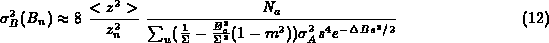These equations show that e.s.u. of atomic parameters depend on completeness, resolution and quality of the data, the completeness and quality of the model and the remaining phase error.

If we replace in equation (11 - 12) B_n with B_{Wilson} and z_n² with <z²> we can get the e.s.u. for an `average' atom in the structure. In principle equations (11 - 12) could be used at any stage of refinement but the derivation used only the diagonal terms of second derivative matrix. Especially in the early stages of refinement off diagonal terms which reflect the interaction between different parameters may also be large.

## 5. Conclusions

1. There is dependence of e.s.u. on B-values as expected, but it is not as simple as substituting the e.s.u. as sqrt{B/8pi²}}
2. There is dependence of e.s.u. on resolution, as expected
3. There is dependence of e.s.u. on completeness of data, as expected
4. There is dependence of e.s.u. on completeness of model, as expected
5. There is dependence of e.s.u. on the quality of data but inclusion of weak data could still improve quality of model. (Sometimes weak data are better than no data)
All equations given here use only diagonal terms of second derivative matrix therefore will give better approximation at high resolution and at the end stages of refinement. These equations do not use restraints.

### References

1. Dodson, E.J., Kleywegt, G.J. & Wilson, K. (1996) Acta Crsyt. D52 228-234
2. Cruickshank, D.W.J. (1949a) Acta Cryst. 2 65-82
3. Cruickshank, D.W.J. (1949b) Acta Cryst. 2 154-157
4. Cruickshank, D.W.J. (1960) Acta Cryst. 13 774-777
5. Cruickshank, D.W.J. (1996) in the Refinement of Macromolecular structures Proceedings of CCP4 Study weekend. pp 11-22
6. Luzzati, V. (1952) Acta Cryst. 5, 802-810
7. Murshudov, G.N., Vagin A.A. & Dodson, E.J. (1997) Acta Cryst. D53 in press
8. Murshudov, G.N., Grebenko, A.I., Brannigan, J.A., Antson, A.A., Barynin, V.V., Dauter, Z., Wilson, K.S. & Melik-Adamyan, W.R. (1997) J.Mol.Biol. in press
9. Read, R.J. (1986) Acta Cryst. A42, 140-149
10. Sheldrick, G.M. (1995) SHELXL-93, a Program for the Refinement of Crystal Structures from Diffraction Data. Institut fuer Anorg.Chemie, Goettingenm Germany.
11. Stuart, A & Ord, K.J. (1991) Kendall's Advanced Theory of Statistics. Vol. 2 5th ed. London, Melbourne, Auckland: Edward Arnold

Figure 1: The parameter for both these distributions has its minimum at 0. The solution for the distribution with second derivative of 2, is more sharply defined than the that with second derivative of 1.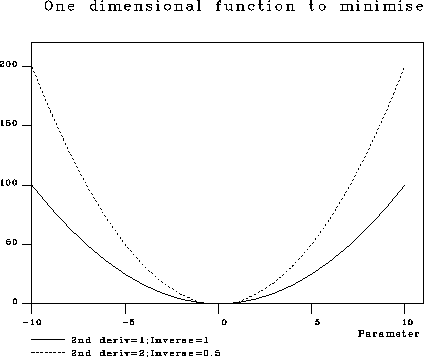Figure 2: B-value dependence of e.s.u. at 1.5A resolution. Dashed lines correspond e.s.u. derived using agreement of `free' reflections, solid lines show e.s.u. derived using agreement of reflections included in refinement. a) e.s.u. for positional parameters. b) e.s.u. for B-values.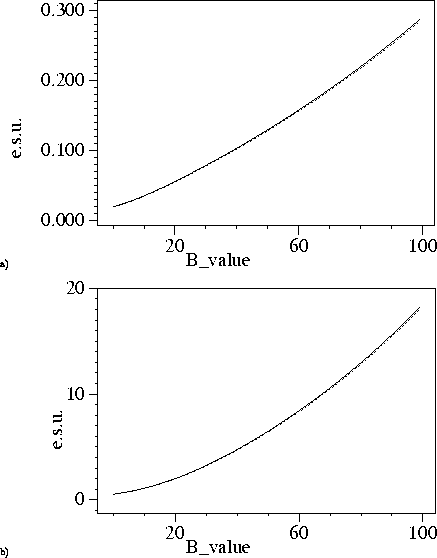Figure 3: B-value dependence of e.s.u. at 1.83A resolution. Dashed lines correspond e.s.u. derived using agreement of `free' reflections, solid lines show e.s.u. derived using agreement of reflections included in refinement. a) e.s.u. for positional parameters. b) e.s.u. for B-values.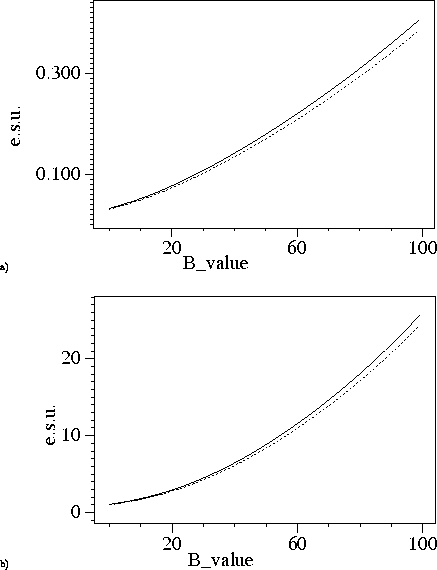Figure 4: B-value dependence of e.s.u. at 1.96A resolution. Dashed lines correspond e.s.u. derived using agreement of `free' reflections, solid lines show e.s.u. derived using agreement of reflections included in refinement. a) e.s.u. for positional parameters. b) e.s.u. for B-values.